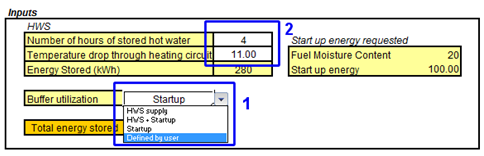Biomass Installation Feasibility ToolThermal Buffer Sizing Manual

Since an exact procedure for sizing the buffer system would provide inaccurate results without knowing the heating circuit and its control configuration, the solution implemented was to supply the user with values and options that may help and give an idea of how big the storage system may be. Additionally, it can even help with deciding how it will be used.

# Inputs

The menu for the inputs is shown below.1 – Buffer utilization: The first decision the user may do is select how he/she plans to use the buffer. Since the two main reasons for having it installed is to supply energy during the start up process and supply hot water during summer (when heating demand usually is very low), not allowing the boiler to work inefficiently in a “on-off” scheme, these are the options offered. A third option, “defined by the user”, was added. The main idea of this part of the tool is to not give a single solution, but allow the user to have a range of possibilities, so he/she can decide which path to take.

The HWS and Defined by user tables are in the same cells. What is shown depends on the selection done with this menu.

2 – Number of hours and temperature variation: If the “defined by user” option is set, the table will ask to input the amount of energy he/she will want to store, in kWh.

If any option that involves HWS is selected, the user will need to input the number of hours that he wants the tank to be able to supply hot water and the temperature drop through the circuit. For two pipe systems this variation is usually around 11 °C and in case of a single pipe it is around 20°C.

## Outputs

There are 3 main calculations done at this part, the first two giving as result the amount of energy necessary for the selected conditions and the last, the amount of water necessary to store this energy.1 - Start-up energy (or thermal lining): The energy that will be used during the start-up process depends basically on two values, size of a boiler (capacity) and moisture content of fuel used. There is no study calculating this energy exactly. Each boiler supplier may provide the user with this information. There is although a “rule of thumb“ that may be used in most cases where the energy required for the thermal lining, in kWh, is numerically correspondent to 50% of the boiler maximum power output, in kW, if the fuel has moisture content up to 40% or, in case of wetter fuels, 70% of the maximum capacity of the boiler.

Using information depending on selected fuel with the fuel comparator and consumption part and the size of the boiler, this energy is calculated.

2 - HWS: The idea here is to define how much energy is necessary to supply hot water for a building, for a given time, based on its mean consumption. The number of hours, defined by the user, is then multiplied by the mean hot water demand, defined by the heat demand model. Using this information, the amount of energy, in kWh, is calculated.

3 - Total Volume of Water: Knowing the total energy to be stored, shown in the “total energy stored” table, it is possible to calculate the volume of water required:Where:

• M is the total mass of water, in kg needed to store the requested energy
• Stored Energy is the energy, in kWh, displayed in “total energy stored table”
• 4.2 is the specific heat of the water in kJ/kg K
• T is the temperature variation in the heating circuit/buffer in K.

Since it 1 litre of water weights 1 kg, the value calculated above is equal to the total volume ( in litres, or dm3) required..

Another important data one must be aware of during the process of observing the thermal buffer size is the effective volume that it will require. Although the steps above resulted in calculating the volume of water needed for a given installation, it does not correspond to the volume that the entire equipment will occupy. One must know the insulation volume, heat exchangers dimensions, etc. in order to have that data available. However, using data from different suppliers, a typical relationship between water volume and total volume was estimated and used (given the number of tanks and total of water needed) to define the individual tank volume. It is important to notice that this relationship is not a strict rule, is just an average of what was observed after investigating data from several suppliers. It just gives an idea of the space taken by the system.